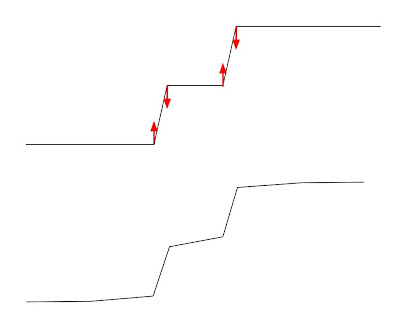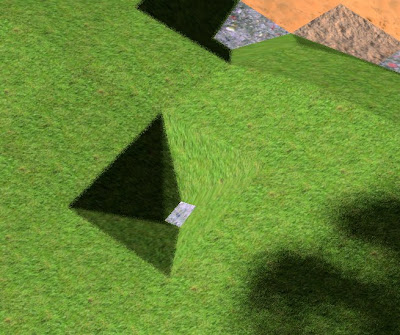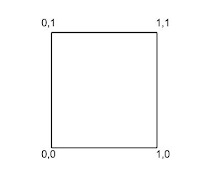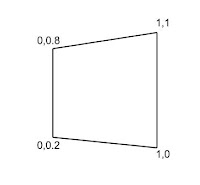## 5 november 2012

### Smooth world from block data

Ephenation is a voxel based world. Everything (almost) are blocks that have a given address. Graphics based on square blocks doesn't look very good. There are ways to make it look good, e.g. the Marching Cubes algorithm.
However, this algorithm has some drawbacks. Every created triangle has a texture, and it is not trivial to decide what texture to use for triangles that span from one block type to another. Another problem is that some cubes in the world shall still be shown as cubes. This leads to difficulties in the transition between smooth terrain and cubistic blocks.

## Use of a filter

In Ephenation, it was decided to use another algorithm, with similarities to a low pass filter. The basic idea is to take every coordinate and add a specific delta to each of the three dimensions. The magnitude of the delta is determined as a function of neighbor blocks. A two dimensional example of this could be:Delta in X dimension
Next, if the same delta is applied in 'y', we get:Delta in Y dimension
The principle is simple; the delta is computed in every dimension and can be applied independently.

## Three dimensions

When computing the delta for 3 dimensions, neighbor vertices has to be take into account from 3 dimensions.

In the figure, there are 8 cubes. They are initially uniformly distributed. The content of these 8 cubes are used to determine the delta of the point P, in the middle, for each of the X, Y and Z dimensions. Only one filter is defined for one dimension, and then the transformation is rotated in three different directions. The algorithm uses a small matrix, of the size 2x2x2, that is initialized with the content of the 8 cubes. The delta computation uses that matrix to determine the delta in one dimension. And then this small matrix is filled with the same content, but rotated for the other dimensions, and the test is repeated.

## Merging normals

After applying the delta, it is possible to change the appearance without changing the geometry. If the normals for all triangles that meet at the same vertex is replaced by an average, then it will further increase the smoothness look of the world. To speed up this process, the vertex data is sorted on vertex position. A std::multiset is used, with pointers to the vertex data. This set of pointers is then sorted. A question is whether normals from different materials shall also be averaged. This is not done currently.

There are some special cases that need to be taken care of. For example, it may be that the sum of the normals is a null vector. When that happens, the normals are simply left unchanged.Non modified normals
And with normals merged, the same geometry will be:Merged normals

## Calibration

The amount of the delta has to be calibrated. See below a video clip where the delta goes from 0 to 0.17.

There is a middle point where the slope is 45 degrees, which corresponds to a calibration constant of 0.125. There is no smooth transition between bitmaps of different kinds. It can be seen in this video clip as a checker pattern. To get smooth transition between different textures is outside of the scope here, and it is not currently implemented in Ephenation.

## Texture mapping

Texture UV mapping in a tiled world is trivial. For example, the front face of a cube can be mapped as shown in the picture.Default UV mapping

When a smoothing filter is applied (coordinates are modified with a delta), it would seem trivial to compute a new UV-mapping. If the new height is decreased from 1 to 0.8 and the lower left is raised by 0.2, then the upper left corner would now be mapped to 0,0.8 instead.Delta applied on left side
However, there may also be delta added to the Z component. Different deltas can be added to the top and bottom. For the left border in the figure above, the height would still be 0.6 units, but the total length of the left border may be longer as it is could be leaning forward in Z. There are extreme cases, where the total height is approaching 0, but the difference in Z for the left side corners are growing dominant. If only X and Y are used for UV mapping, then the bitmap will appear stretched. This has not been taken care of yet.The red material is stretched

## Chunk borders

In Ephenation, all blocks are organized into chunks. A chunk consists of 32x32x32 blocks organized in a matrix. With a given chunk, it is easy to find individual blocks, as well as adjacent blocks.. But when analyzing blocks at the chunk border, data have to be fetched from another chunk. To simplify this process, the list of neighbor chunks are first prepared. This part of the algorithm is not complete yet, and the border between chunks can be seen as having no delta applied.

## Random noise

There is yet another way to improve the realism, and that is to add a random value to the delta. 2D simplex noise is used to add a random noise to the height. The drawback with 2D noise that do not use the height component, is that it will generate the same height delta for all coordinates with the same horizontal coordinates. But that is an acceptable simplification, as it won't be noticeable unless floors above each other are compared. The drawback with 3D simplex noise is that it is more costly.

Special care has to be taken for some materials, like water. It would not look natural to have permanent slopes and hills in the water.

## Performance

All together, it is quite a lot of computation that is done to every chunk. With a long viewing distance, a lot of chunks are needed. A thread pool is used for this, using available cores on the CPU. Still, with a 1 or 2 core CPU, the cost can be too high.

## Update history

1.2.